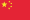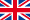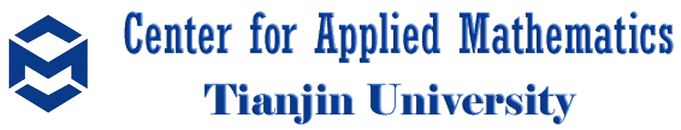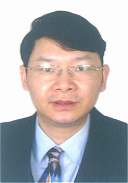中文English## Professors

 William Y.C. Chen Yuping Duan Zaihui Gan Kathy Q. Ji Renjin Jiang Kangwei Li Jinghai Shao Xiaotao Sun Fengyu Wang Gengsheng Wang Ou Wu Yifei Wu Yong Zhang Chuanming Zong

 Hua Chen Peter Paule

## Associate Professors

 Song Dai Xiequan Fan Ling He Eryan Hu Huaiqian Li Zhenling Peng Ruipeng Shen Baofang Song WenYi Tian Yaohong Wang Huaming Wu Tian Xu Xun Yu Haixiang Zhang

## Assistant Professors

 Xin Chen Yingjun Deng Alan J.X. Guo Haoyang Huang Xing Huang Cong Liang Wenjun Ma Bo Ning Bin Wei Jie Wu Manting Xie Song Yang Yubiao Zhang Mingfeng Zhao Mingshuo Zhou Fei Zhu

## Post-Doctors

 Lingyan Cheng Teng Fang Chao Ji Hongying Lin Jijian Song Dazhao Tang Lixia Wang Jiakuan Xu Xu Zhang

 Albert Y. Liu Boran Li### Email: wangfy@tju.edu.cn

Research Interests
• Functional inequalities and applications

• stochastic analysis on Riemannian manifolds

• infinite-dimensional stochastic analysis

• stochastic (partial) differential equations

Education
1983.09-1987.07, B.S, Mathematics, Anhui Normal University, China

1987.09-1990.07, M.Sc., Probability Theory and Statictics, Beijing Normal University, China

1990.09-1993.07, Ph.D, Probability Theory and Statictics, Beijing Normal University, China

Selected Books
 F.-Y. Wang, Functional Inequalities, Markov Semigroups and Spectral Theory, Science Press. 2005.

 F.-Y. Wang, Harnack Inequality and Applications for Stochastic Partial Differential Equations, Springer, 2013.

 F.-Y. Wang, Analysis of Diffusion Processes on Riemannian Manifolds, World Scientific, 2014.
Selected Publications
 F.-Y. Wang, Sharp explicit lower bounds of heat kernels, Ann. Probab. 25 (1997)

 F.-Y. Wang, Harnack inequalities for log-Sobolev functions and estimates of log-Sobolev constant, Ann. Probab. 27(1999)

 M. F. Chen and F.-Y. Wang, Cheeger's inequalities for general symmetric forms and existence criterion for spectral gap, Ann. Probab. 28(2000)

 M. Cranston and F.-Y. Wang, Equivalence of coupling and shift-coupling, Ann. Probab. 28(2000)

 F.-Y. Wang, Gradient estimates of Dirichlet semigroups and applications to isoperimetric inequalities, Ann. Probab. 32 (2004)

 F.-Y. Wang, Harnack inequality and applications for stochastic generalized porous media equations, Ann. Probab. 35(2007)

 F.-Y. Wang, Log-Sobolev inequalities: different roles of Ric and Hess, Ann. Probab. 37(2009)

 F.-Y. Wang, Harnack inequality for SDE with multiplicative noise and extension to Neumann semigroup on non-convex manifolds, Ann. Probab. 39(2011)

 F.-Y. Wang, Integration by parts formula and shift Harnack inequality for stochastic equations, Ann. Probab. 42(2014)

 F.-Y. Wang Integrability Conditions for SDEs and Semi-linear SPDEs, Ann. Probab.. (accepted)

 M. F. Chen and F.-Y. Wang, On order-preservation and positive correlations for multidimensional diffusion processes, Probab. Theory Relat. Fields 95(1993)

 F.-Y. Wang, Application of coupling method to the Neumann eigenvalue problem, Probab. Theory Relat. Fields 98(1994)

 F.-Y. Wang, Estimates of the first Dirichlet eigenvalues by using diffusion processes, Probab. Theory Relat. Fields 101(1995)

 F.-Y. Wang, On estimation of logarithmic Sobolev constant and gradient estimates of heat semigroups, Probab. Theory Relat. Fields 108(1997)

 F.-Y. Wang, Logarithmic Sobolev inequalities on noncompact Riemannian manifolds, Probab. Theory Relat. Fields 109(1997)

 M. F. Chen, F.-Y. Wang, Estimation of the first eigenvalue of second order elliptic operators, J. Funct. Anal. 131(1995)

 M. F. Chen, F.-Y. Wang, Estimates of logarithmic Sobolev constant: an improvement of Bakry-Emery criterion, J. Funct. Anal. 144(1997)

 A. Thalmaier, F.-Y. Wang, Gradient estimates for harmonic functions on regular domains in Riemannian manifolds, J. Funct. Anal. 155(1998)

 F.-Y. Wang, Functional inequalities for empty essential spectrum, J. Funct. Anal. 170(2000)

 M. Rockner, F.-Y. Wang, Weak Poincare inequalities and convergence rates of Markov semigroups, J. Funct. Anal. 185(2001)

 F.-Y. Wang, Functional inequalities and spectrum estimates: the infinite measure case, J. Funct. Anal. 194 (2002)

 M. Rockner, F.-Y. Wang, Harnack and functional inequalities for generalized Mehler semigroups, J. Funct. Anal. 203 (2003)

 F.-Y. Wang, Probability distance inequalities on Riemannian manifolds and path spaces, J. Funct. Anal. 206 (2004)

 E. Priola and F.-Y. Wang, Gradient estimates for diffusion semigroups with singular coefficients, J. Funct. Anal. 236(2006)

 F.-Y. Wang, A Harnack-type inequality for Non-Symmetric Markov Semigroups, J. Funct. Anal . 239（2006）

 F.-Y. Wang, Second fundamental form and gradient of Neumann semigroups, J. Funct. Anal. 256(2009)

 G. Da Prato, M. Rockner, F.-Y. Wang, Singular stochastic equations on Hilbert spaces: Harnack inequalities for their transition semigroups, J. Funct. Anal. 257(2009)

 P. Cattiaux, A. Guillin, F.-Y. Wang, L. Wu, Lyapunov conditions for Super Poincaré inequalities, J. Funct. Anal. 256(2009)

 S. Feng, W. Sun, F.-Y. Wang, F. Xu, Functional inequalities for the unlabeled two-parameter infinite-alleles diffusion, J. Funct. Anal. 260(2011)

 F.-Y.Wang, Criteria on spectral gap of Markov operators, J. Funct. Anal. 266(2014)

 F.-Y. Wang, L. Xu, X. Zhang, Gradient estimates for SDEs driven by multiplicative Levy noise, J. Funct. Anal.269(2015), 3195--3219.

 M. F. Chen, F.-Y. Wang, Estimation of spectral gap for elliptic operators, Trans. Amer. Math. Soc. 349:3(1997)

 V. I. Bogachev, M. Rockner, F.-Y. Wang, Elliptic equations for invariant measures on finite and infinite dimensional manifolds, J. Math. Pure Appl. 80(2001)

 D. Bakry, M. Ledoux and F.-Y. Wang, Perturbations of functional inequalities using growth conditions, J. Math. Pure Appl. 87(2007)

 F.-Y. Wang, From super Poincare to weighted log-Sobolev and entropy-cost inequalities, J. Math. Pure Appl. 90(2008)

 F.-Y. Wang, Harnack inequalities on manifolds with boundary and applications, F.-Y. Wang, Harnack inequalities on manifolds with boundary and applications, J. Math. Pures Appl. 94(2010)

 F.-Y. Wang, X. Zhang, Derivative formula and applications for degenerate diffusion semigroups, J. Math. Pures Appl. 99(2013)

 V.I. Bogachev, M. Rockner, M., F.-Y. Wang, Invariance implies Gibbsian: some new results, Comm. Math. Phys. 248 (2004)

 J. Ren, M. Rockner, F.-Y. Wang, Stochastic generalized porous media and fast-diffusion equations, J. Diff. Equations 238(2007)

 M. Rockner, F.-Y. Wang, Non-monotone stochastic generalized porous media equations, J. Diff. Equations 245(2008)

 A. Guillin, F.-Y. Wang, Degenerate Fokker-Planck Equations : Bismut Formula, Gradient Estimate and Harnack Inequality, J. Diff. Equations 253(2012)

 F.-Y. Wang, Gradient Estimates and Applications for SDEs in Hilbert Space with Multiplicative Noise and Dini Continuous Drift, J. Diff. Equations 260 (2016)

 F.-Y. Wang, Log-Sobolev inequality on non-convex manifolds, Advances in Math. 222(2009)

Center for Applied Mathematics, Tianjin University, No.92 Weijin Road, Tianjin 300072, P. R. China